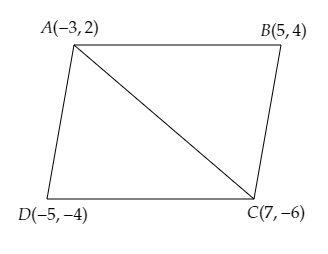# Find the area of a quadrilateral $ABCD$, the coordinates of whose vertices are $A (-3, 2), B (5, 4), C (7, -6)$ and $D (-5, -4)$.

Given:

Vertices of the quadrilateral $ABCD$ are $A(-3, 2), B(5, 4), C(7, -6)$ and $D(-5, -4)$.

To do:

We have to find the area of the quadrilateral.

Solution:

Join $A$ and $C$ to get two triangles $ABC$ and $ADC$.This implies,

Area of quadrilateral $ABCD=$ Area of triangle $ABC+$ Area of triangle $ADC$.

We know that,

Area of a triangle with vertices $(x_1,y_1), (x_2,y_2), (x_3,y_3)$ is given by,

Area of $\Delta=\frac{1}{2}[x_{1}(y_{2}-y_{3})+x_{2}(y_{3}-y_{1})+x_{3}(y_{1}-y_{2})]$

Therefore,

Area of triangle $ABC=\frac{1}{2}[-3(4+6)+5(-6-2)+7(2-4)]$

$=\frac{1}{2}[-3(10)+5(-8)+7(-2)]$

$=\frac{1}{2}[-30-40-14]$

$=\frac{1}{2} \times (-84)$

$=42$ sq. units.

Area of triangle $ADC=\frac{1}{2}[-3(-6+4)+7(-4-2)+-5(2+6)]$

$=\frac{1}{2}[-3(-2)+7(-6)+(-5)(8)]$

$=\frac{1}{2}[6-42-40]$

$=\frac{1}{2} \times (-76)$

$=38$ sq. units.

Therefore,

The area of the quadrilateral $ABCD=42+38=80$ sq. units.

The area of the quadrilateral $ABCD$ is $80$ sq. units.

Updated on: 10-Oct-2022

39 Views